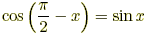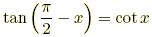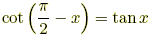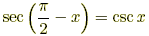index: click on a letter A B C D E F G H I J K L M N O P Q R S T U V W X Y Z A to Z index index: subject areas numbers & symbols sets, logic, proofs geometry algebra trigonometry advanced algebra & pre-calculus calculus advanced topics probability & statistics real world applications multimedia entrieswww.mathwords.com about mathwords website feedback

Cofunction Identities

Trig identities showing the relationship between sine and cosine, tangent and cotangent, and secant and cosecant. The value of a trig function of an angle equals the value of the cofunction of the complement of the angle.

 Cofunction Identities, radians Cofunction Identities, degreessin (90° – x) = cos x cos (90° – x) = sin xtan (90° – x) = cot x cot (90° – x) = tan xsec (90° – x) = csc x csc (90° – x) = sec x